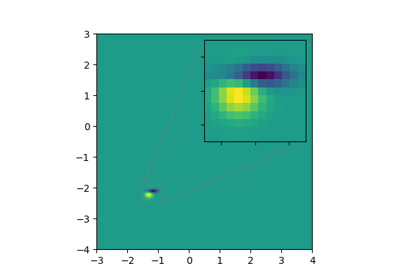matplotlib.axes.Axes.inset_axes¶

Axes.inset_axes(self, bounds, \*, transform=None, zorder=5, \*\*kwargs)[source]

Add a child inset axes to this existing axes.

Parameters: bounds[x0, y0, width, height]Lower-left corner of inset axes, and its width and height. transformTransformDefaults to ax.transAxes, i.e. the units of rect are in axes-relative coordinates. zordernumberDefaults to 5 (same as Axes.legend). Adjust higher or lower to change whether it is above or below data plotted on the parent axes. **kwargsOther keyword arguments are passed on to the Axes child axes. axThe created Axes instance.

Warning

This method is experimental as of 3.0, and the API may change.

Examples

This example makes two inset axes, the first is in axes-relative coordinates, and the second in data-coordinates:

fig, ax = plt.subplots()
ax.plot(range(10))
axin1 = ax.inset_axes([0.8, 0.1, 0.15, 0.15])
axin2 = ax.inset_axes(
[5, 7, 2.3, 2.3], transform=ax.transData)

Examples using matplotlib.axes.Axes.inset_axes¶